# Development and Validation of Sampling Plans for the European Red Mite Panonychus Ulmi (Acari: Tetranychidae)

London Journal of Research in Science: Natural and Formal
Volume | Issue | Compilation
Authored by Said OUASSAT , NA
Classification: NA
Keywords: binomial and sequential sampling plan, monitoring protocol, tetranychidae, sampling unit, crop protection, RVSP.
Language: English
A total of 24 data sets was available from April until September 2018 to develop a binomial sequential sampling plan to monitor Panonychus ulmi population. Action threshold of 2.31 mobile forms /sample unit was determined using the empirical model and used for developing and evaluating sequential sampling plans through 500 Monte Carlo iterations. Based on data of presence /absence sampling plan, a fixed precision sequential plan at D= 0.20; 0.25; 0.30; 0.35 and 0.50 was established. The mean sample numbers decrease significantly with increasing precision using 500 iterations. Presence of a common k (k c ) of negative binomial distribution was verified and its value was calculated according to Bliss and Owen’s procedure. For Wald’s sequential probability ratio test, a minimum of 19 sample units is required to detect the presence of harmless populations of P. ulmi, comparatively to 4 units obtained by Iwao’s sequential sampling plan, which is an alternative to overcoming α and β error values ​​and does not need any mathematical distribution of P. ulmi. The terminology and methodology of each plan were clarified and the results were discussed. Developing a reliable sampling method for monitoring P. ulmi is essential for implementing integrated pest management measures.

# Development and validation of sampling plans for monitoring the European red mite, Panonychus ulmi (Acari: Tetranychidae)

## ____________________________________________

ABSTRACT

A total of 24 data sets was available from April until September 2018 for developing sampling plans  to monitor the Panonychus ulmi population. Action threshold of 2.31 mobile forms /sample unit was determined using the empirical model, which based on the presence or absence of one or more mite per sample unit. The mean-variance relationship was established, and the threshold mite density was used for developing Green’s model at various precision levels (D = 0.20, 0.25, 0.30, 0.35 and 0.50), the sequential probability ratio test of Wald, and Iwao’s model. All established sequential plans were thereafter evaluated through 500 Monte Carlo iterations. Result of Green’s plan showed that the mean sample numbers decrease significantly with increasing precision. For Wald’s model, the presence of a common k (kc) of negative binomial distribution was verified, and its value is calculated according to Bliss and Owen’s method. Results showed that a  minimum of 19 sample units is required to detect the presence of harmless populations of P. ulmi, comparatively to 4 units obtained by Iwao’s sequential sampling plan, which is an alternative to overcoming α and β error values ​​and does not need any mathematical distribution of P. ulmi. The terminology and methodology of each plan were clarified, and the results are discussed. Developing a reliable sampling method for monitoring P. ulmi is essential for implementing integrated pest management measures.

Keywords: binomial and sequential sampling plan, monitoring protocol, Tetranychidae, sampling unit, crop protection, RVSP

Author: Laboratory of Health and Environment, Crop protection research unit, Faculty of Sciences, Moulay Ismail University, 11201, Zitoune, Meknes, Morocco.

1. INTRODUCTION

The European red spider mite, Panonychus ulmi (Koch, 1836) (Acari: Tetranychidae), is a significant damaging mite pest  of apple at lower population densities. The damage closely resembles magnesium deficiency, bright-yellow patches develop on the leaflets, and gradually coalesce until the whole leaf withers and die (Foster and Barker, 1978).

Development and reproduction s of P. ulmi have been studied under controlled conditions. The previously published results reported the effect of temperature on development and life history parameters (Northcraft and Watson, 1987; Qui and Li, 1988; Zhou et al., 2006; Zou et al., 2018), this species develops and reproduces rapidly at high temperatures and can close a considerable number of generations during the growing season. In front of severing attacks of P. ulmi, acaricides are sprayed frequently during the growing season to limit decreasing the phytosanitary status of crops (Plaut and Cohen, 1967).

Panonychus ulmi can develop resistance to miticides and cross-resistance to other pesticides when it has developed resistance to one pesticide (Dağlı and Tunç, 2001). It is recommended often to use integrated pest management programs wherever possible (Mollet and Sevacherian, 1984; Çakmak et al., 2005). The spray program for other pests and diseases should be designed to have the minimum disruptive effect on predatory mites in biological control cases (Sarwar, 2013).

An aspect of any pest management program is having a sampling procedure that allows determination of when a pest population is so abundant, as to require control. Most of these protocols consist of at least two items: a threshold pest density, which, when exceeded by a local population, dictates management intervention (usually a pesticide application), and a reliable and efficient method for estimating or classifying pest abundance. Much work devoted to devising and analyzing sampling methods that-maximize precision while minimizing sampling costs (Kuno, 1991; Binns & Nyrop, 1992). Counting all mites on a single sample unit often takes an hour or more. Consequently, few researchers or pest control advisors are willing to commit the time necessary for whole sampling plants for mites (Wilson and Room, 1983).

In this document, we develop binomial and sequential sampling plans that can be used to efficiently monitor P. ulmi through time, and how the performance of any plan was assessed by the use of resampling for validation of sequential plan program (RVSP). We describe the methods by specifying in a general way what it is designed to do for establishing the constituents of each plan. Then we discuss the results and compare the efficiency of each monitoring protocol of P. ulmi with the potential effect of some parameters on the performance of each procedure.

1. # MATERIAL AND METHODS

2.1  Sampling data

The data have been collected on Jeromine variety. The area of the sampled plot is 1.5 ha. Fifty sampling positions were selected, and 100 apple leaves are harvested weekly from April to September 2018. A total of 24 data sets was available for statistical and modelling analysis. Field data were recorded as a proportion of the infested sample unit and mean density of Panonychus ulmi during each sampling occasion.

2.2  Fixed-sample-size plan:

The binomial sampling plan based on data of the presence or absence of one or more P. ulmi per sample unit has been developed. This empirical model is practically simple, and convenient for monitoring the mite pest populations without the need of any mathematical distribution. The density- infestation level relationship established by using Nachman’s function (Nachman, 1984), help in determining theoretically the economic threshold corresponding to mite density considered as an intervention limit. The value of the economic threshold will be used to develop sequential sampling plans.

2.3  Fixed-precision sampling plan

The sequential sampling plan of Green (1970) was developed from the model of  Kuno (Kuno, 1969). The mean-variance relationship is fitted by using Taylor’s power law:(Taylor, 1961), a and b, respectively are the regression intercept and regression slope. Both parameters were expressed under logarithmic in the formulae given by Southwood (1978).In this plan, a decision to take additional sample units should be terminated when the defined precision level was achieved. The fixed-precision stop line was calculated as:Where Tn is the cumulative density of P. ulmi, a and b are respectively, Taylor’s regression parameters. D is the fixed-precision, and n is the sample size. D was expressed as the ratio of the standard error of the mean to mean (D = SEM/mean) at precision levels of 0.20, 0.25, 0.30, 0.35, and 0.50.

2.4 Sequential probability ratio test (SPRT) of Wald (1945)

The Wald’s SPRT test (Wald, 1945) is based on two hypotheses instead to three hypothesis procedure developed by Nyrop et al. (1994) for tripartite classification of mite pest density, the null hypothesis (H0: x = x0) against the alternative hypothesis (H1: x=x1) (x0 < xt < x1}), where xt is the economic threshold estimated from fixed-size sampling plan, x0 and xt are parameters that are used to define an acceptable SPRT (Binns and Nyrop, 1992). Stop lines for sequential sampling plan can be computed by using predetermined lower and upper limits of economic thresholds (x0 and x1) and risk levels for making type I (α) (probability of accepting the alternative hypothesis when the null hypothesis is correct) and type II (β) errors (probability of accepting the null hypothesis when the alternative hypothesis is correct). Wald’s sequential sampling plan is defined by its operating characteristic (OC) and average sample number (ASN) of observations needed to make a classification given any mean value. The OC and ASN functions were computed from 500 Monte Carlo simulations and the dispersion parameter k of the negative binomial distribution calculated at economic threshold according to the method of Bliss and Owen (1958).

2.5  Iwao sequential sampling plan

Iwao's method based on the relationship of Lloyd's (1967) index of mean crowding (x*) to m. Iwao (1968) showed that the regression of (x*) on m is linear and described by:.   α (intercept) is termed the index of basic contagion and describes the component of the population. β (slope) is termed the density-contagiousness coefficient related to the distribution pattern of these components within the habitat, with values of β >1 representing an aggregated distribution, β = 1, and β < 1 corresponding to a random, and regular distribution, respectively. The stabilized variance (s2) was given by Iwao and Kuno (1968):. Where m = mean, while α and β are parameters of the relationship. α and β were calculated by plotting mean crowding (x*) on mean densities (m).

The method developed by Iwao, 1968 (1975) for distributions exhibiting a linear relationship for the regression of x* on m should be used.  The upper (TU) and lower (TL) limits of the plan were calculated from:Where N = number of samples taken; ET = Economic Threshold; t = Student's t value at the chosen level of significance of a test bilateral for an infinite number of degrees of freedom; α=contagion index (intercepted) and β = (slope) or distribution coefficient.

The maximum number of samples required to decide  if the critical density remains between the upper and lower limits, and is given by:. With D is the precision level.

The performance of the sequential sampling plans must be evaluated through computing probability curves and average sample numbers ASN from 500 Monte Carlo simulations. We have used Resampling for the Validation Sampling plan software Program (RVSP) (Naranjo and Hutchison, 1997), R (3.4.3) program for statistical adjustment tests, and Excel 2013 for graphing.

1. # RESULTS AND DISCUSSION

3.1 Variance-mean relationships:

Because the variance increased significantly faster with the mean densities, since it is normal to having the high variance values in aggregative distribution case, the variance was stabilised using Taylor (s2=amb) and Iwao models (s2 = (α+1)m+(β-1)m2). Means and variances from the 0.22 -9.23 P. ulmi per sample unit were fit to a straight line by using Taylor's power law (Figure 2) and Iwao’s regression (Figure 8). For both models, the equations respectively are : ( log(s2) = 0.30+1.14log(m); a = 0.30, b =1.14, r2 = 0.97, dl = 22, P < 0.05))  and  (x* = 1.10m + 0.9, α = 0.007, β = 1.10, r2 = 0.99, dl = 22, P < 0.05). The slope values greater significantly than 1 attesting that the pattern dispersion of P. ulmi is aggregative.

Based on the values of r2 for both models, such comparison is only valid if the variables and scale transformations are the same, and the number of parameters of the models is the same (Kvålseth, 1985). The same data sets were used to predict variance, and a significant correlation has been observed. Or, even though the r2 values were similar, the functional relationship was very different for each, because the dependent variables are not the same and the data scales are different (Kvålseth, 1985; Scott and Wild, 1991).

3.2  Fixed-sample-size plan

The theoretical values of mean densities corresponding to infestation levels ​​as well as confidence and prediction intervals were given in Table 1.

Table 1: Relationship between the proportion of infested sample units and densities of P. ulmi according to Nachman function with confidence (CI) and prediction intervals (PI) to 95%

 Panonychus ulmi Infestation level Mean density ± CI ± Pl 0.10 1.02 0.96-1.11 0.37-2.90 0.20 1.94 1.74-2.17 0.68-5.32 0.30 2.31 2.10-2.54 0.82-6.48 0.40 3.59 3.28-4.01 1.28-10.07 0.50 4.63 3.88-4.89 1.55-12.23 0.60 5.24 4.90-6.67 2.39-14.31 0.70 6.20 5.46-7.05 2.21-17.44 0.80 7.86 6.82-8.89 3.55-19.23 0.90 9.23 5.41-10.12 5.21-23.44

The relationship between P. ulmi density and proportion of infested sample units was established through linear regression, the values ​​of statistical parameters are obtained by plotting mean densities on infestation level (a = 1.64, b = 0.78, r2 = 0.91, d1 = 22. F = 63.142, P < 0.05) (Figure 1).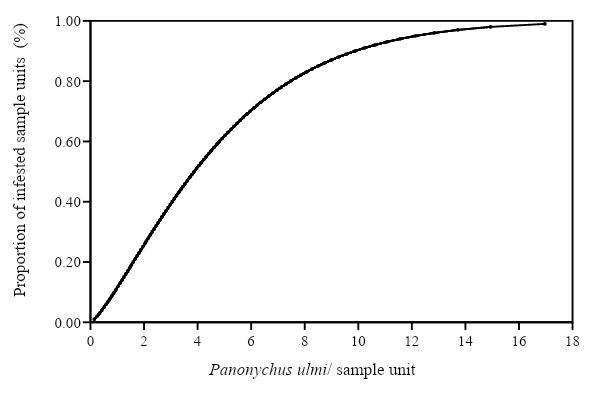Figure 1: Binomial sampling stop line plan and theoretical relationship between the proportion of infestation level and the mean density of P. ulmi obtained by using Nachman’s model.

Based on 24 data sets, the Fixed-sample-size sampling plan or positive binomial was developed first without considering a theoretical distribution of mite (Poisson, binomial, and negative binomial distributions). The Fixed-sample-size sampling plan is an empirical model overcoming the calculation of common k (kc) when the distribution of mites conforms to negative binomial law (Anscombe, 1950). The relationship between densities of P. ulmi and the proportion of infested sample units was established using Nachman function, allowing to grant theoretically at each infestation level a density value within confidence and prediction intervals at-risk level of 5% (Nyrop et al., 1989). Empirical model using Nachman's function corrects the variations related to the proportion of infested sample units and mean density changes due to interactions with potential zoological and natural systems in the presence of natural enemies, for example.

A significant correlation was observed between mean densities and infestation level (r2 =0.91, P < 0.05). The proportion of infested sample units increased slowly with an increase in mean densities (Figure 1). The densities of less than two mites do not exceed a proportion of 25 %, while about 90 % of leaves were infested at an average density of 9.23 P. ulmi / sample unit. The mean density of 2.31 P. ulmi/ sample unit relative to 30 % of infestation and α value of 0.05 was used to develop and to valid sequential sampling plans.

Our results can be compared with those of several previous studies using empirical models (Croft et al., 1976; Gerrard & Chaing, 1970; Wilson & Room, 1983; Hilbert & Logan, 1983; Opit et al., 2003). Although the predatory mite, Typhlodromus (T.) setubali (Dosse, 1961), is present in the sampled plot, we found that the aggregation of the P. ulmi population was not greatly affected. Or, in biological control of mite pests, the mean densities and associated variances and the kc value can be varied during the season according to their spatial distribution (Anscombe, 1950; Shaw et al., 1998; Giles et al., 2000; Nyrop et al., 1994).

3.3  Fixed-precision sampling plan

Based on Taylor’s power law, the log variance against log means regression showed that the variance increased significantly with mean density (r2 = .97, P < 0.05) (Figure 2). Intercept value (a) was 0.30. The slope (b) value of 1.14 greater than one, indicating that the dispersion pattern of the P. ulmi population is aggregative (P < 0.05).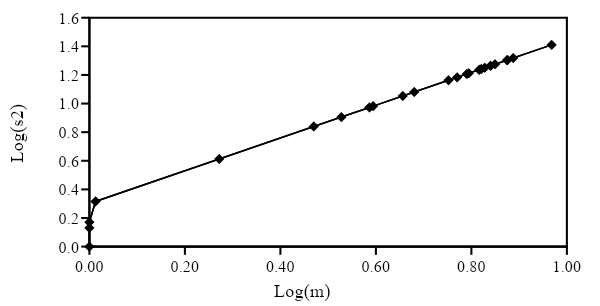Figure 2: Mean-variance relationship using Taylor power law. Taylor's model was estimated by using log (s2) = log(a)+blog(m).

Green’s fixed-precision stop lines for estimating the density at D = 0.20; 0.25; 0.30; 0.35 and 0.50 are given in Figure 3. These stop lines show that fewer samples were needed for estimating high densities, and more samples were needed for estimating low densities. RVSP simulation with field data showed that densities > 2 P. ulmi/leaf at a fixed precision of 0.20 could be estimated with 20 sample units (Table 2). A different trend is observed at a fixed precision of 0.25, 0.30, 0.35, and 0.50, where the number of sample units needed decreases respectively to 13, 11, and 10 leaves. However, the density of 2.31 mite/leaf could be estimated with 20 sample units, while more than 20 leaves are needed for estimating densities < 2.31 at fixed precision of 0.20 and much more for low densities. Generally, the average sample number decreases inversely with fixed-precision values. The range of P. ulmi densities that can be estimated with 20 sampling units changes according to the level of precision chosen. At the fixed- precision of 0.50 and 0.35, 20 sample units are enough to estimate densities ranging from 0.7 to 9.29 mites/leaf and 1.03 to 9.29, respectively, whereas at 0.20, 0.25 and 0.30, more sample units were needed for estimating densities less than 1.87mite pest/ leaf.

The observed precision in estimating P. ulmi densities of 0.22-9.29 mites was ≤ 0.20 when D = 0.20 for 21 of 24 data sets. At the fixed precision of 0.25, 0.30, and 0.35, the observed D was ≤ these precision levels for 22 of 24 data sets. Finally, at the fixed precision of 0.50, the observed precision was ≤ 0.50 for all data sets. Observed precision was at or below the fixed precision in cases where the sample variance for the field data sets was equal to or less than the variance predicted by Taylor’s power law because this law did not predict sample variance of field data at low mean densities.Figure 3: Sequential sampling graph for P. ulmi. Both Tn (cumulative densities) and n (average sample number) are logarithmic scales. Stop lines are calculated for precision levels (D) of 0.20, 0.25, 0.30, 0.35 and 0.50.

Table 2: Performance of Green's fixed-precision sequential sampling plan for estimating mean densities of P. ulmi at fixed-precision levels of D = 0.20, 0.25, 0.30, 0.35, and 0.50

 Data set MeanA VarianceB Predicted varianceC Fixed precision D D=0.20 D=0.25 D=0.30 D=0,35 D=0,50 ND DE ND DE ND DE ND DE ND DE 1 0,22 0,430 0,353 187 0,21 122 0,26 85 0,30 64 0,35 33 0,48 2 0,71 1,279 1,351 69 0,19 44 0,24 32 0,28 24 0,33 13 0,44 3 0,77 1,755 1,483 65 0,21 42 0,26 29 0,31 22 0,36 13 0,47 4 1,03 2,110 2,069 50 0,20 32 0,24 23 0,29 18 0,34 11 0,42 5 1,87 2,556 4,098 30 0,11 19 0,14 14 0,17 11 0,19 10 0,20 6 2,95 7,555 6,908 20 0,17 13 0,21 11 0,24 10 0,24 10 0,24 7 3,86 9,988 9,400 17 0,19 11 0,22 10 0,23 10 0,23 10 0,23 8 4,53 12,965 11,292 14 0,15 10 0,18 10 0,19 10 0,19 10 0,19 9 5,65 13,271 14,544 12 0,12 10 0,13 10 0,13 10 0,13 10 0,14 10 6,23 16,878 16,267 11 0,10 10 0,11 10 0,11 10 0,10 10 0,11 11 5,89 14,668 15,254 11 0,15 10 0,16 10 0,16 10 0,16 10 0,16 12 7,51 21,254 20,150 10 0,16 10 0,17 10 0,16 10 0,16 10 0,17 13 6,92 19,233 18,347 11 0,15 10 0,16 10 0,16 10 0,16 10 0,16 14 7,07 20,456 18,804 10 0,09 10 0,10 10 0,10 10 0,10 10 0,10 15 7,49 19,265 20,089 10 0,13 10 0,13 10 0,13 10 0,13 10 0,13 16 9,29 33,784 25,710 10 0,16 10 0,16 10 0,16 10 0,16 10 0,16 17 7,72 25,658 20,797 10 0,14 10 0,15 10 0,15 10 0,14 10 0,15 18 6,55 14,145 17,228 11 0,15 10 0,15 10 0,15 10 0,15 10 0,14 19 6,17 16,564 16,088 11 0,16 10 0,16 10 0,16 10 0,17 10 0,16 20 6,73 18,541 17,771 11 0,18 10 0,19 10 0,19 10 0,19 10 0,19 21 6,62 16,666 17,439 11 0,17 10 0,16 10 0,17 10 0,17 10 0,17 22 4,79 14,887 12,038 14 0,19 10 0,22 10 0,23 10 0,23 10 0,23 23 3,93 6,422 9,596 16 0,15 11 0,18 10 0,19 10 0,20 10 0,20 24 3,37 5,856 8,046 18 0,14 12 0,17 10 0,19 10 0,19 10 0,19

A: Sample means, expressed as the mean number of mobile forms of Panonychus ulmi. Each mean number based on 100 sample units.

B: Sample variance of field data.

C: The predicted sample variance is calculated as amb, where a = 1.14, b = 0.30, and m is the sample mean.

D: The mean number of sample units required to estimate m as determined by the RVSP software.

E: Observed mean precision level for estimating m as determined by the RVSP software.

The sequential sampling design of Green (1970) for estimating populations at fixed-precision D = 0.20, 0.25, 0.30, 0.35, and 0.50 was developed and validated by using 500 Monte Carlo simulations (Naranjo and Hutchison, 1997). Virtually, A reasonable D is usually between 0.20 and 0.35 (Southwood, 1978; Hutchison et al., 1988). For all densities observed from April to September, precision levels of 0.30, 0.35, and 0.50 offer the possibility of taking sample sizes below 100 sample units for classifying the densities of P. ulmi; this number increases when the precision is 0.20 (Table 2). A similar  ASN result was obtained by Mollet et al. (1984) at D = 0.20, 0.25, and 0.30 with significant correlations for both mean-variance relationships (r2 = 0.95 for Taylor and 0.80 for Iwao’s regression).

To further monitor and manage the population of P. ulmi, similar plans have been established for different life stages, including eggs, larvae, nymphs, and adults. This approach shows that, when data sets were used for validation, the resulting sequential sampling design can be used reliably to ensure that the desired fixed precision levels are achieved (O’Rourke and Hutchison, 2003; Serra et al., 2013).

3.4  Wald’s sequential probability ratio test (SPRT)

The linear regression equation (plotting y' on x’) is: y '= 0.63x’+0.10 (r2 = 0.98: dl = 22, P < 0.05). The intercept value (0.10) is not significantly different from zero (P > 0.05) (Figure 4A). Therefore, the condition of the negative binomial law is verified. The presence of a common k (kc) is obvious (Bliss and Owen, 1958).

To calculate the common k (kc), we plotted on the mean densities of Panonychus ulmi. The linear regression equation is y = 0.09x+2.64 (r2 = 0.88, dl = 22, P < 0.05). The regression slope is not significant at the error level of 5 % and shows no trend existing (Figure 4B). The basic postulate of the Wald’s procedure is respected, and the presence of a common k is justified. The calculation of kc value corresponds to an approximate estimate using the formula:which gives a kc value equal to 1.58.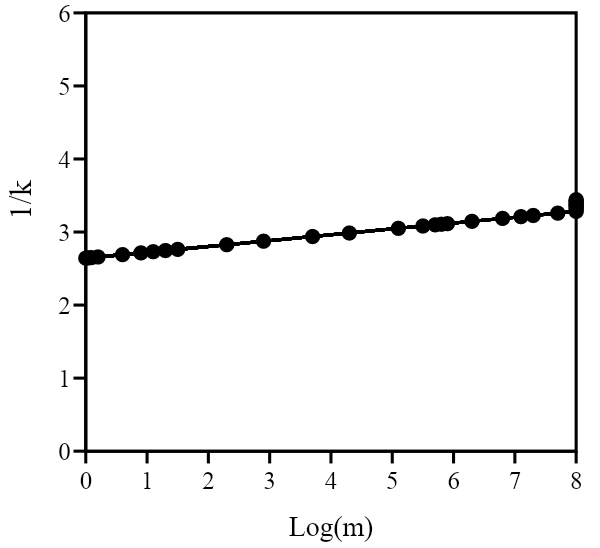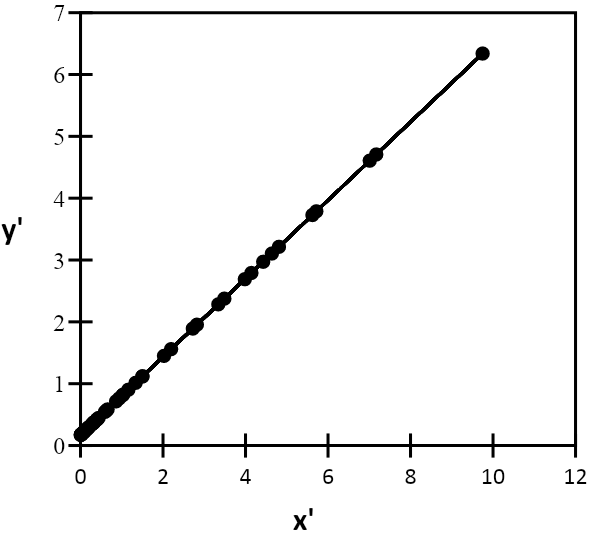(A)                                                                                      (B)

Figure 4: Regression estimate of a common k (kc) for P. ulmi (A). Relation of the 1/kc to the mean based on 24 sample data (B).

The lower and upper stop lines for classifying the infestation level with respect to the ET of 2.31 P. ulmi/ sample unit are shown in Figure 5. The lower stop line intersects the x-axis at 19 sample units. Therefore, a minimum of 19 sample units must be examined for classifying the infestation level. The accuracy of classifying the P. ulmi infestation levels relative to an AT was tested by RVSP at tally threshold T = 0 using the 24 independent data sets. The OC function was near unity when m < 2.14. At a mean density value of 2.26 (x1), the OC function was 0.90. At an m value of 2.36 (x2), the OC function was 0.20. At m > 2.36, the OC function decreased from 0.20 to zero. At an m value of 2.31 (ET) (Figure 6). The OC curve showed that the actual error rate in classifying infestation as being x1 when, in fact, it is above x2 (β error) was 0.50.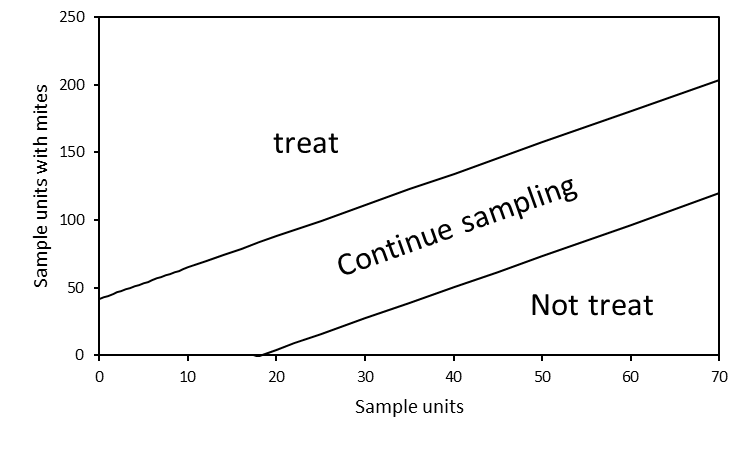Figure 5: The stop lines of SPRT for classifying the mean densities of P. ulmi relative to an economic threshold (ET).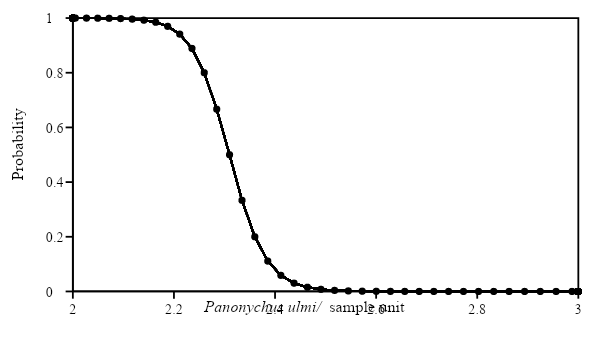Figure 6: Operating characteristic (OC) curve for the sequential probability ratio test sampling plan (α = β = 0.2, x1 = 2.26, x2 = 2.36, kc = 1.58)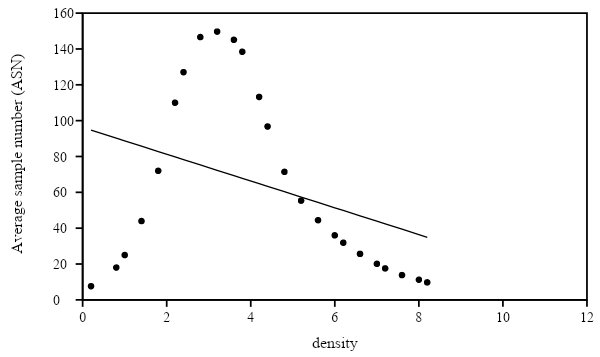Figure 7: Average sample number (ASN) curve for the sequential probability ratio test sampling plan (α = β = 0.2; x1 = 2.26; x2 = 2.36; kc = 1.58).

The ASN curve showed that for classifying the range densities of 0.22-9.29, about 8-149 sample units are needed with more samples for classifying the mean density at the ET of 2.31mites per leaf. Each of the 24 independent data sets used to evaluate the performance of the sequential sampling plan was based on 100 sample units. Therefore, with 100 sample units, mean densities of ≤ 2.14 or ≥ 4.12 can be correctly classified on average concerning the fixed ET. However, for mean densities of Panonychus ulmi between 2.14 and 4.12, we can expect that more than 100 sample units will be required (Figure 7). The uncertainty in classifying the mean densities of P. ulmi increases near the threshold. Therefore, the ASN is near the ET. More sample units needed to be examined to cross either the upper or lower stop line when P. ulmi density was between x1 and x2 than when it was less than x1 or than x2. Uncertainty of classifying mite density at the ET results in a large number of samples being required to reach a decision (Binns, Nyrop, Werf, & Werf, 2000). A pest mite control measure should be applied when the mean density or infestation level is at or above the economic threshold. The use of these sequential sampling plans in integrated pest management programs for pests will result in the application of pest control measures only when needed (Morris, 1960; Binns and Nyrop, 1992; Binns, 1994).

Several methods of calculating kc have been discussed (Pedigo and Buntin, 1994) and  several estimates of a common k have been described (Beall, 1942; Kleczkowski, 1949; Anscombe, 1949a; 1949b; 1950; Bliss & Fisher, 1953), but  a simple method based on the use of linear regression to calculate kc is often used (Bliss and Owen, 1958)

The stability of the parameter k is not always present. The parameter k tends to increase with the increase of the mean, and its biological interpretation is not obvious (Pedigo and Buntin, 1994; Robinson and Smyth, 2008). Ruesink suggests the use of Taylor’s power law when the average density of sampled populations varies by more than one order of magnitude (Ruesink, 1980). If there is a k, the Wald procedure can be used to develop a sequential sampling plan.

Using Bliss & Owen's (1958) model, the kc value was 1.58. Generally, kc of the negative binomial distribution closes to 2. The high kc corresponds to the Poisson distribution. Wald’s sequential sampling is evaluated by examining the operating characteristic (OC) and average sample number (ASN) functions (Nyrop et al., 1999). The OC curve describes the probability of making a no intervention decision (crossing the lower decision boundary) as a function of the mean pest density. A k value of 1.58, The OC curve is flatter and increases the classification accuracy of the plan (the smaller type I and type II error rates) (Nyrop et al., 1994). The ASN curve indicates the expected number of samples required to decide on as a function of the mean pest density. Generally, for Wald’s sequential probability ratio test, a flatter OC function means less robustness and precision of the sampling plans. A flatter ASN function means that fewer samples are required to classify pest population density.

3.5  Iwao sequential sampling plan

The linear regression equation between mean crowding and mean density of P. ulmi is x* = 1.10m + 0.9 (r2 = 0.99, dl =22, P < 0.05) (Figure 8). The value of the contagion index corresponds to the intercept (0.90), and the value of the distribution coefficient corresponds to the slope of the regression (1.10).

At an error level of 5 %, and D = 0.50, the equations of the non-parallel stop lines of the sequential sampling plan developed according to Iwao procedure are: y = 2.31x + 1.71(4.89x)1/2 and y = 2.31x-1.71(4.89x)1/2 for the upper and lower limits, respectively (Figure 9). The same rules apply concerning the decision to process (value above the upper limit) or not (value below the lower boundary) or to continue sampling (the middle zone between the upper and lower limits).

A minimum number of 4 samples is necessary to cross the negative zone of the lower limit, whereas a maximum number of 57 sample units are needed to determine whether the population level is equal to the economic threshold at a confidence interval predetermined.Figure 8: Mean-variance relationship using  Iwao's model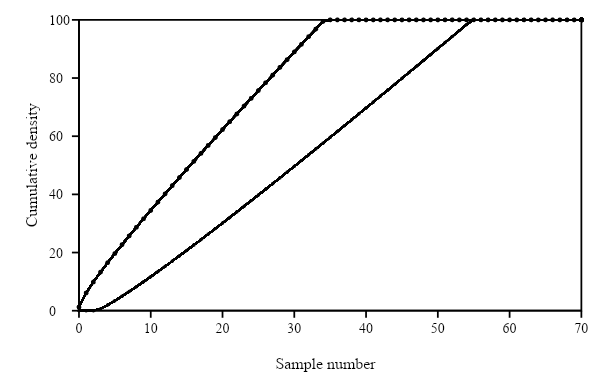Figure 9: Acceptance curves of a sequential sampling plan according to the  Iwao procedure for classifying the mean densities of P. ulmi relative to an economic threshold (ET).

Table 3: Sequential decision table, giving the cumulative number of P. ulmi required for a decision from 4 to 20 sample units with an error level of 0.05.

 Sample number lower threshold upper threshold 4 0 16 5 3 20 6 5 23 7 7 26 8 8 29 9 10 31 10 12 34 11 13 37 12 15 40 13 17 43 14 19 46 15 21 48 16 22 51 17 24 54 18 26 57 19 28 59 20 30 62

The failure to establish a k value from the sampling data precluded the use of traditional sequential sampling methods. In Figure 8, the regression of mean crowding (x*) on mean density (m) accounts for 99 % of the variation in the distribution of P. ulmi. The mathematical relationship between the mean density m and the mean crowding (x*) describes some characteristics of the spatial distribution inherent in a species in a given habitat. Iwao (1968) has shown that this relationship is often linear and described by a simple regression line. Two parameters describe the type of spatial distribution of the mite. These are the contagion index α and the slope β, appointed distribution coefficient. The first of these parameters characterize the basic unit of the population, while the second describes the distribution of these basic units in space. The validity of the regression was checked using the correlation coefficient (r2) (Montgomery et al., 2012).

After calculating the upper and lower decision limits by using the formulas supplied by (Iwao, 1975), equations were fit to the data by the least-squares method. The resulting graphs (Figure 9) remind one of the typical sequential sampling graphs, except that the lines are not parallel. For pest management use, scouts prefer a table such as Table 3.

The Wald’s and Iwao’s sequential sampling plans are different in terms of the minimum number of samples required to leave the negative zone and the economic threshold used for decision making. The developed Wald’s (1945) sequential plan is more restrictive since a minimum of 19 samples (Figure 5) is necessary to detect the presence of harmless populations of the mite compared to 4 samples for the sequential plan developed with the Iwao’s procedure. The thresholds of the Iwao Plan seem more permissive as to the cumulative number of P. ulmi per sample unit required before deciding to intervene or not.

1. CONCLUSIONS

The sequential sampling plans presented here are powerful tools for monitoring and managing the mite pest populations. Sampling needs an efficient procedure to estimate the damage related to pest mites and phytophagous insects and succeed integrated management programs in orchards. Sequential sampling protocols based on three hypothesis and calculated probabilities could be a quick method for making control decisions in biological control against mite populations by phytoseiidae and requires additional research on various aspects of predator-prey relationships. Population dynamics studies are also needed to transfer the action threshold from 2.31 mobile forms per leaf to the number of eggs, ie, how many eggs per sample unit must be observed to produce later an infestation of 2.31 mite pest per leaf?

It is possible to envisage the integration of a simultaneous sampling of different insect pests to make the sampling profitable, thus allowing the application of sequential plans for plant protection. The sequential sampling plans may be easier to apply over large areas, where the time and cost may be reduced by adequate sampling. In this context, current practices in arboriculture will need to be revised and focused more on sampling. The aggregative behavior of pest mites in small areas to be sampled seems to be the cause of the difficulty of applying a sequential design that minimizes the sampling effect.

Although sampling to classify the dynamic and distribution of the pest mites population, is generally expensive and involves the time and money, sampling remains essential for monitoring population dynamics and decision-making. However, sampling costs could be offset by an informed decision based on established concepts and developed sampling plans. The choice of an effective method is, therefore, crucial when the objective is to reduce sampling effort and associated costs.

ACKNOWLEDGMENTS

The authors would like to thank the anonymous reviewers for their valuable comments on this article and declare no conflict of interest.

BIBLIOGRAPHY

1. Anscombe, F.J. 1949a. The Statistical Analysis of Insect Counts Based on the Negative Binomial Distribution. Biometrics 5(2): 165–173.
2. Anscombe, F.J. 1949b. Large-Sample Theory of Sequential Estimation. Biometrika 36(3-4): 455–458.
3. Anscombe, F.J. 1950. Sampling Theory of the Negative Binomial and Logarithmic Series Distributions. Biometrika 37(3-4): 358–382.
4. Beall, G. 1942. The Transformation of Data from Entomological Field Experiments so that the Analysis of Variance Becomes Applicable. Biometrika 32(3-4): 243–262.
5. Binns, M.R. 1994. Sequential sampling for classifying pest status. In Handbook of Sampling Methods for Arthropods in Agriculture. 1st Edition, CRC Boca Raton. 137-174 pp.
6. Binns, M.R., Nyrop, J.P. 1992. Sampling Insect Populations for the Purpose of IPM Decision Making. Annual Review of Entomology 37(1): 427–453.
7. Binns, M.R., Nyrop, J.P., Werf, W. van der., Werf, W. 2000. Sampling and Monitoring in Crop Protection: The Theoretical Basis for Developing Practical Decision Guides. CABI. 300 pp.
8. Bliss, C.I., Fisher, R.A. 1953. Fitting the Negative Binomial Distribution to Biological Data. Biometrics 9(2): 176–200.
9. Bliss, C.I., Owen, A.R.G. 1958. Negative Binomial Distributions with a Common k. Biometrika 45(1-2): 37–58.
10. Çakmak, İ., Başpinar, H., Madanlar, N. 2005. Control of the Carmine Spider Mite Tetranychus cinnabarinus Boisduval by the Predatory Mite Phytoseiulus persimilis (Athias-Henriot) in Protected Strawberries in Aydın, Turkey. Turk J Agric For 29(4): 259–265.
11. Croft, B.A., Welch, S.M., Dover, M.J. 1976. Dispersion Statistics and Sample Size Estimates for Populations of the Mite Species Panonychus ulmi and Amblyseius fallacis on Apple. Environ Entomol 5(2): 227–234.
12. Dağlı, F., Tunç, Í. 2001. Dicofol resistance in Tetranychus cinnabarinus: resistance and stability of resistance in populations from Antalya, Turkey. Pest Management Science 57(7): 609–614.
13. Foster, G.N., Barker, J. 1978. A New Biotype of Red Spider Mite (Tetranychus urticae (Koch)) causing Atypical Damage to Tomatoes. Plant Pathology 27(1): 47–48.
14. Gerrard, D.J., Chaing, H.C. 1970. Density Estimation of Corn Rootworm Egg Populations Based upon Frequency of Occurrence. Ecology 51(2): 237–245.
15. Giles, K.L., Royer, T.A., Elliott, N.C., Kindler, S.D. 2000. Development and validation of a binomial sequential sampling plan for the greenbug (Homoptera: Aphididae) infesting winter wheat in the southern plains. J. Econ. Entomol. 93(5): 1522–1530.
16. Green, R.H. 1970. On fixed precision level sequential sampling. Res Popul Ecol 12(2): 249–251.
17. Hilbert, D.W., Logan, J.A. 1983. Empirical Model of Nymphal Development for the Migratory grasshopper, Melanoplus sanguinipes (Orthoptera: Acrididae) 1. Environmental Entomology 12(1): 1–5.
18. Hutchison, W.D., Hogg, D.B., Poswal, A.M., Berberet, R.C., Cuperus, G.W. 1988. Implications of the Stochastic Nature of Kuno’s and Green’s Fixed-Precision Stop Lines: Sampling Plans for the Pea Aphid (Homoptera: Aphididae) in Alfalfa as an Example. J Econ Entomol 81(3): 749–758.
19. Iwao, S. 1968. A new regression method for analyzing the aggregation pattern of animal populations. Res Popul Ecol 10(1): 1–20.
20. Iwao, S. 1975. A new method of sequential sampling to classify populations relative to a critical density. Res Popul Ecol 16(2): 281–288.
21. Iwao, S., Kuno, E. 1968. Use of the regression of mean crowding on mean density for estimating sample size and the transformation of data for the analysis of variance. Res Popul Ecol 10(2): 210–214.
22. Kleczkowski, A. 1949. The Transformation of Local Lesion Counts for Statistical Analysis. Annals of Applied Biology 36(1): 139–152.
23. Kuno, E. 1969. A new method of sequential sampling to obtain the population estimates with a fixed level of precision. Res. Popul. Ecol. 11(2): 127–136.
24. Kuno, E. 1991. Sampling and Analysis of Insect Populations. Annual Review of Entomology 36(1): 285–304.
25. Kvålseth, T.O. 1985. Cautionary Note about R 2. The American Statistician 39(4): 279–285.
26. Lloyd, M. 1967. Mean Crowding. Journal of Animal Ecology 36(1): 1–30.
27. Mollet, J.A., Sevacherian, V. 1984. Pesticide and Seasonal Effects on Within-Plant Distribution of Tetranychus cinnabarinus (Boisduval) (Acarina: Tetranychidae) in Cotton. J Econ Entomol 77(4): 925–928.
28. Mollet, J.A., Trumble, J.T., Walker, G.P., Sevacherian, V. 1984. Sampling Scheme for Determining Population Intensity of Tetranychus cinnabarinus (Boisduval) (Acarina: Tetranychidae) in Cotton. Environ Entomol 13(4): 1015–1017.
29. Montgomery, D.C., Peck, E.A., Vining, G.G. 2012. Introduction to Linear Regression Analysis. John Wiley & Sons. 680 pp.
30. Morris, R.F. 1960. Sampling Insect Populations. Annu. Rev. Entomol. 5 (1): 243–264.
31. Nachman, G. 1984. Estimates of Mean Population density and spatial distribution of Tetranychus urticae (Acarina: Tetranychidae) and Phytoseiulus persimilis (Acarina: Phytoseiidae) Based upon the proportion of empty sampling units. Journal of Applied Ecology 21(3): 903–913.
32. Naranjo, S.E., Hutchison, W.D. 1997. Validation of Arthropod Sampling Plans Using a Resampling Approach: Software and Analysis. Am Entomol 43(1): 48–57.
33. Northcraft, P.D., Watson, T.F. 1987. Developmental biology of Tetranychus cinnabarinus (Boisduval) under three temperature regimes. Southwestern Entomologist 12(1): 45–50.
34. Nyrop, J.P., Agnello, A.M., Kovach, J., Reissig, W.H. 1989. Binomial sequential classification sampling plans for European red mite (Acari: Tetranychidae) with special reference to performance criteria. J Econ Entomol 82(2): 482–490.
35. Nyrop, J.P., Binns, M.R., van der Werf, W., Kovach, J. 1994. Cascading tripartite binomial classification plans to monitor European red mite (Acari,Tetranychidae) through a season; development and evaluation of a new methodology for pest monitoring. Exp Appl Acarol 18(3): 123–153.
36. Nyrop, J.P., Binns, M.R., van der Werf, W. 1999. Sampling for IPM Decision Making: Where Should We Invest Time and Resources? Phytopathology 89(11): 1104–1111.
37. Opit, G.P., Margolies, D.C., Nechols, J.R. 2003. Within-Plant Distribution of Twospotted Spider Mite, Tetranychus urticae Koch (Acari: Tetranychidae), on Ivy Geranium: Development of a Presence-Absence Sampling Plan. J Econ Entomol 96(2): 482–488.
38. O’Rourke, P.K., Hutchison, W.D. 2003. Sequential sampling plans for estimating European corn borer (Lepidoptera: Crambidae) and corn earworm (Lepidoptera: Noctuidae) larval density in sweet corn ears. Crop Protection 22(7): 903–909.
39. Pedigo, D.P.M., Buntin, L.P. 1994. Handbook of sampling methods for arthropods in agriculture. Statistics for Describing Populations. CRC Press, Boca Raton. Florida. 33-54 pp.
40. Plaut, H.N., Cohen, M. 1967. The two-spotted (Carmine) Spider Mite (Tetranychus cinnabarinus Boisd.) on Apple in Israel. Zeitschrift Für Angewandte Entomologie 60(1-4): 460–466.
41. Qui, F., Li, Q.S. 1988. Biology and population dynamics of Tetranychus cinnabarinus (Boisduval) on cotton. Insect Knowledge 25(6): 333–338.
42. Robinson, M.D., Smyth, G.K. 2008. Small-sample estimation of negative binomial dispersion, with applications to SAGE data. Biostatistics 9(2): 321–332.
43. Ruesink, W.G. 1980. Introduction to Sampling Theory. In Sampling Methods in Soybean Entomology, M. Kogan, and D.C. Herzog. Springer: New York. 61–78 pp.
44. Sarwar, M. 2013. Management of spider mite Tetranychus cinnabarinus (Boisduval) (Tetranychidae) infestation in cotton by releasing the predatory mite Neoseiulus pseudo longispinosus (Xin, Liang and Ke) (Phytoseiidae). Biological Control 65(1): 37–42.
45. Scott, A., Wild, C. 1991. Transformations and R 2. The American Statistician 45(2): 127–129.
46. Serra, G.V., Porta, N.C.L., Avalos, S., Mazzuferi, V. 2013. Fixed-Precision Sequential Sampling Plans for Estimating Alfalfa Caterpillar, Colias lesbia, Egg Density in Alfalfa, Medicago sativa, Fields in Córdoba, Argentina. J Insect Sci 13(1). Article 41.
47. Shaw, D.J., Grenfell, B.T., Dobson, A.P. 1998. Patterns of macroparasite aggregation in wildlife host populations. Parasitology 117(6): 597–610.
48. Southwood, T.R.E. 1978. Ecological methods: with particular reference to the study of insect populations. Chapman and Hall. London. 22 pp.
49. Taylor, L.R. 1961. Aggregation, variance and the mean. Nature 189(4766): 732-735.
50. Wald, A. 1945. Sequential Tests of Statistical Hypotheses. The Annals of Mathematical Statistics 16(2): 117–186.
51. Wilson, L.T., Room, P.M. 1983. Clumping Patterns of Fruit and Arthropods in Cotton, with Implications for Binomial Sampling. Environmental Entomology 12(1): 50–54.
52. Zhou, Y., Piao, C., Qiu, G., Zhang, P., Meng, W., Fang, H. 2006. Life Table for 3 Mite Species under Different Temperature. Journal of Shenyang Agricultural University 37(2): 173–176.
53. Zou, Z., Xi, J., Liu, G., Song, S., Xin, T., and Xia, B. 2018. Effect of temperature on development and reproduction of the carmine spider mite, Tetranychus cinnabarinus (Acari: Tetranychidae), fed on cassava leaves. Experimental and Applied Acarology 74(4): 383–394.#### For Authors

/symposiums/webinars, networking opportunities, and privileged benefits.
Authors may submit research manuscript or paper without being an existing member of LJP. Once a non-member author submits a research paper he/she becomes a part of "Provisional Author Membership".

Know more#### For Institutions

Society flourish when two institutions come together." Organizations, research institutes, and universities can join LJP Subscription membership or privileged "Fellow Membership" membership facilitating researchers to publish their work with us, become peer reviewers and join us on Advisory Board.

Know more#### For Subscribers

Subscribe to distinguished STM (scientific, technical, and medical) publisher. Subscription membership is available for individuals universities and institutions (print & online). Subscribers can access journals from our libraries, published in different formats like Printed Hardcopy, Interactive PDFs, EPUBs, eBooks, indexable documents and the author managed dynamic live web page articles, LaTeX, PDFs etc.

Know more